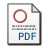# A Review of Student Test Properties in Condition of Multifactorial Linear Regression

by Pavelescu, Florin Marius
Published in Romanian Journal of Economic Forecasting
, 2009, volume 10 issue 1, 63-75Requires a PDF viewer such as Xpdf or Adobe Acrobat Reader  250Kb

## Abstract

Having in view previous contributions of the author related to the impact of collinearity on the estimated values of parameters of multifactorial linear regressions, in this paper the correlation between Student and Fisher test is emphasized and a correction of the standard computation of the Student test is proposed, in order to increase the respective test relevance and to detect the occurrence of “statistical illusions” determined by collinearity. Also, the impact of adding a new explanatory variable in the linear regression equation is analyzed and the conditions in which such a step is efficient are determined.

Keywords: Correlation between Fisher test and Student test computed values, Coefficient of alignment to collinearity hazard, Corrected Sudent Test, Interdependence between adjusted coefficient of determination and corrected Sudent Test, Anticollinearity
JEL Classification:
C51, C52